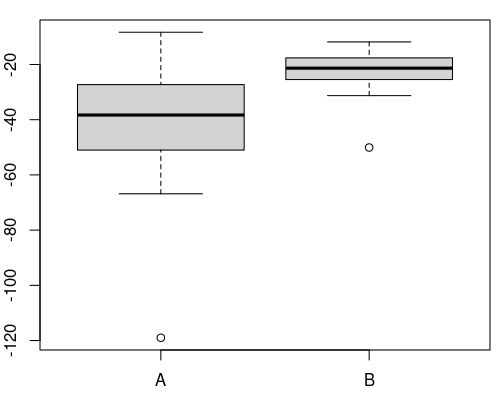## Exam 1

1. #### Question

In the following figure the distributions of a variable given by two samples (A and B) are represented by parallel boxplots. Which of the following statements are correct? (Comment: The statements are either about correct or clearly wrong.)1. The location of both distributions is about the same.
2. Both distributions contain no outliers.
3. The spread in sample A is clearly bigger than in B.
4. The skewness of both samples is similar.
5. Distribution A is right-skewed.

#### Solution

1. False. Distribution B has on average higher values than distribution A.
2. False. There are observations which deviate more than 1.5 times the interquartile range from the median.
3. True. The interquartile range in sample A is clearly bigger than in B.
4. True. The skewness of both distributions is similar, both are about symmetric.
5. False. Distribution A is about symmetric.